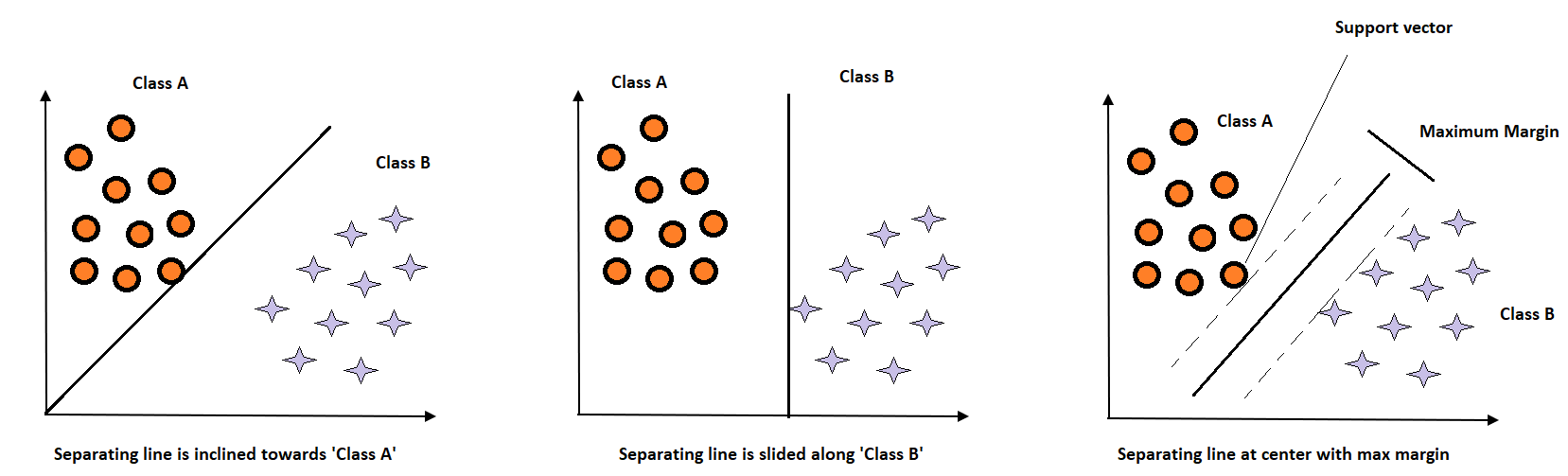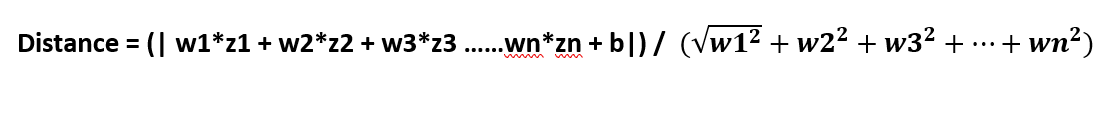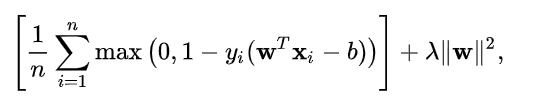# Day 44(ML) — Support Vector Machine(Supervised Learning)

Support Vector Machine or SVM is a supervised learning algorithm, capable of handling data that exhibit complex functions when compared to Linear regression and Logistic regression. The basic working principle is to find the maximum margin between the categories. The concept can be well understood with a picture.Fig 1 — shows various boundary lines

As seen from the above diagram, the objective is to create a maximum margin between two classes ‘A’ and ‘B’. If the margin is very close to either one of the categories, then a new test data with a small shift away from the margin will be misclassified. So an optimal margin that is equidistant to both the targets would be a desirable one. We will be using the linear algebraic concepts discussed earlier. So, it’s time to recollect the vector dot product, the magnitude of vector and unit vector.

Table of contents:

• Maths behind SVM
• Hard margin and Soft margin
• Kernel trick for non-linear separable data
• Pros & Cons

Maths behind SVM: This is the interesting part where we get to know how the algorithm actually works. But before that, let’s understand a few terminologies from linear algebra.

• Hyperplane — From the above example, we have given a separating line(1D). If the data points exist in two-dimensional space, then the boundary would a line. The equation of the hyperplane(separator) is w1x1 + w2x2 +…. + wnxn + b = 0. This is similar to the equation of a straight line but rewritten for higher-dimensional inputs.
• Distance between a point & a hyperplane — Since the objective is to find the maximum distance between the support vector(a point)and the hyperplane, we need the formula for calculating that distance. As mentioned earlier the equation of the hyperplane is w1x1 + w2x2 +…. + wnxn + b = 0 and the point is (z1,z2,z3…zn) (i.e) the support vector.The formula for the distance between a point and a hyperplane

Note: Strongly recommend to watch the video on how to find the distance between a point and hyperplane.

The overall objective is to find the minimum-maximum distance (i.e) minimum refers to the support vector that is close to the boundary/separator and maximum indicates, to maximize the space between the SV & the hyperplane. The whole condition can be written as,

Finding the distance w.r.t (w1,w2,w3) such that it produces the max(min(distance)). Since we have considered a binary classification problem.

w1x1 + w2x2 +…. + wnxn + b > 0 for positive class

w1x1 + w2x2 +…. + wnxn + b < 0 for negative class

Usually, in classification problems, we consider the target as ‘0’ and ‘1’. But for SVM, we will keep the output as +1 and -1. When we multiply the actual value with the predicted, then the resulting output should always be a positive value. Because if both predicted and the actual are positive then result will be +ve, the same logic goes for if the two values are negative. What if the model misclassified the data?. The resultant will be negative.

yn = actual value

w1x1 + w2x2 + ….wnxn + b = predicted value

yn * (w1x1 + w2x2+ …. + wnxn + b) is +ve for correct predictions

yn * (w1x1 + w2x2+ …. + wnxn + b) is -ve for misclassifications

Substituting this in the above distance equation, we have:

Since the value of actual * predicted = 1, we want to maximize 1 / magnitude of W (L2 norm). This could also be rewritten as minimum(magnitude of W (L2 norm).

Hard margin and Soft margin: The above-derived formula corresponds to a perfectly linearly separable data (i.e) hard margin. But in reality, there is always overlap in the distributions between the categories. To make the model more flexible, we go with a soft margin. Instead of having a flawless boundary, we allow a few misclassifications to accommodate the distribution mix. For such scenarios, the maximized formula is tweaked to include the hinge loss(number of misclassified data). The goal of the optimization is to reduce,The formula for softmargin from wiki

If the data is perfectly classified, then the first term will give zero which corresponds to the hard margin. Conversely, for misclassified data, the result will be proportional to how far the data point lies away from the intended location. Lambda is a hyperparameter, which allows the flexibility in the soft margin. If the value is too high, then more penalty is issued for misclassification so the model tries to find the perfect margin(overfitting) and if it too low, then it would result in underfitting.

Kernel trick for non-linear separable data: Sometimes the data we receive cannot be linearly separable at all. Recollecting the polynomial function we used for linear regression. Applying a similar trick here called Kernel trick to convert the non-separable data into separable one by transforming the data into a higher order. For instance, take the rbf(radial basis function).

Pros of SVM:

• SVM’s are very good when we have no idea about the data.
• It works well even with unstructured data such as texts.
• The kernel trick is one of the main advantages of SVM. As it can accommodate non-separable data as well.
• Overfitting can be controlled by proper choice fo hyperparameters.

Cons of SVM:

• Since the complexity of the algorithm is high, the whole training process is time-consuming.
• The overall computational cost is comparatively high.
• SVM will not produce better outcomes when the distribution of the target classes are mixed to a higher degree.

Recommended Reading:

https://www.analyticsvidhya.com/blog/2020/10/the-mathematics-behind-svm/

## Nerd For Tech

From Confusion to Clarification

## Nerd For Tech

NFT is an Educational Media House. Our mission is to bring the invaluable knowledge and experiences of experts from all over the world to the novice. To know more about us, visit https://www.nerdfortech.org/. Don’t forget to check out Ask-NFT, a mentorship ecosystem we’ve started

Written by

## Nandhini N

AI Enthusiast | Blogger✍## Nerd For Tech

NFT is an Educational Media House. Our mission is to bring the invaluable knowledge and experiences of experts from all over the world to the novice. To know more about us, visit https://www.nerdfortech.org/. Don’t forget to check out Ask-NFT, a mentorship ecosystem we’ve started

## More From Medium

Medium is an open platform where 170 million readers come to find insightful and dynamic thinking. Here, expert and undiscovered voices alike dive into the heart of any topic and bring new ideas to the surface. Learn more

Follow the writers, publications, and topics that matter to you, and you’ll see them on your homepage and in your inbox. Explore

If you have a story to tell, knowledge to share, or a perspective to offer — welcome home. It’s easy and free to post your thinking on any topic. Write on Medium